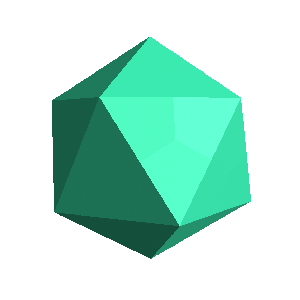Up: The Archimedean Solids

# The Icosahedron, Dodecahedron, and the IcosidodecahedronIcosahedron 20 triangular faces12 verticesDodecehedron 12 pentagonal faces20 verticesIcosidodecahedron 20 triangular faces12 pentagonal faces

If, when truncating the vertices of either the icosahedron or the dodecahedron, we move in exactly half way, the result is the icosidodecahedron. Thus, we can think of the icosidodecahedron as being the limiting case of either the truncated icosahedron or the truncated dodecahedron.

This happens because the icosahedron and dodecahedron are dual solids. Notice that the icosahedron has 20 faces and 12 vertices, while the dodecahedron has 12 faces and 20 vertices. Other Platonic solids which are duals are the cube and the octahedron--see the cuboctahedron page. The tetrahedron is self-dual; it has 4 vertices and 4 faces.

Next: What happens when you truncate the icosidodecahedron?
Go back to The Archimedean Solids